# An introduction to frequent subgraph mining

In this blog post, I will give an introduction to an interesting data mining task called frequent subgraph mining, which consists of discovering interesting patterns in graphs. This task is important since data is naturally represented as graph in many domains (e.g. social networks, chemical molecules, map of roads in a country). It is thus desirable to analyze graph data to discover interesting, unexpected, and useful patterns, that can be used to understand the data or take decisions.

What is a graph? A bit of theory…

But before discussing the analysis of graphs, I will introduce a few definitions.  A graph is a set of vertices and edges, having some labels. Let’s me illustrate this idea with an example. Consider the following graph: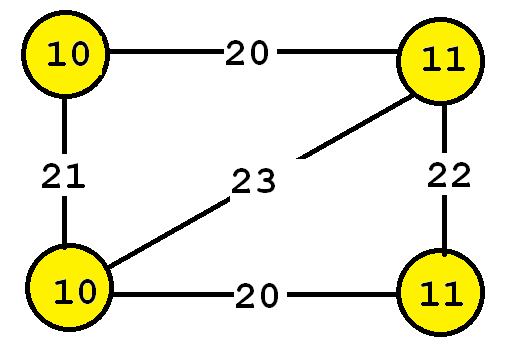This graph contains four vertices (depicted as yellow circles). These vertices have labels such as “10” and “11”.  These labels provide information about the vertices. For example, imagine that this graph is a  chemical molecule. The label 10 and 11 could represent the chemical elements of Hydrogen and Oxygen, respectively. Labels do not need to be unique. In other words, the same labels may be used to describe several vertices in the same graph. For example, if the above graph represents a chemical molecule, the labels “10” and “11” could be used for all vertices representing Oxygen and Hydrogen, respectively.

Now, besides vertices, a graph also contains edges. The edges are the lines between the vertices, here represented by thick black lines.  Edges also have some labels. In this example, four labels are used, which are 20, 21, 22 and 23.  These labels represents different types of relationships between vertices. Edge labels do not need to be unique.

Types of graphs: connected and disconnected

Many different types of graphs can be found in real-life. Graphs are either connected or disconnected.  Let me explain this with an example. Consider the two following graphs: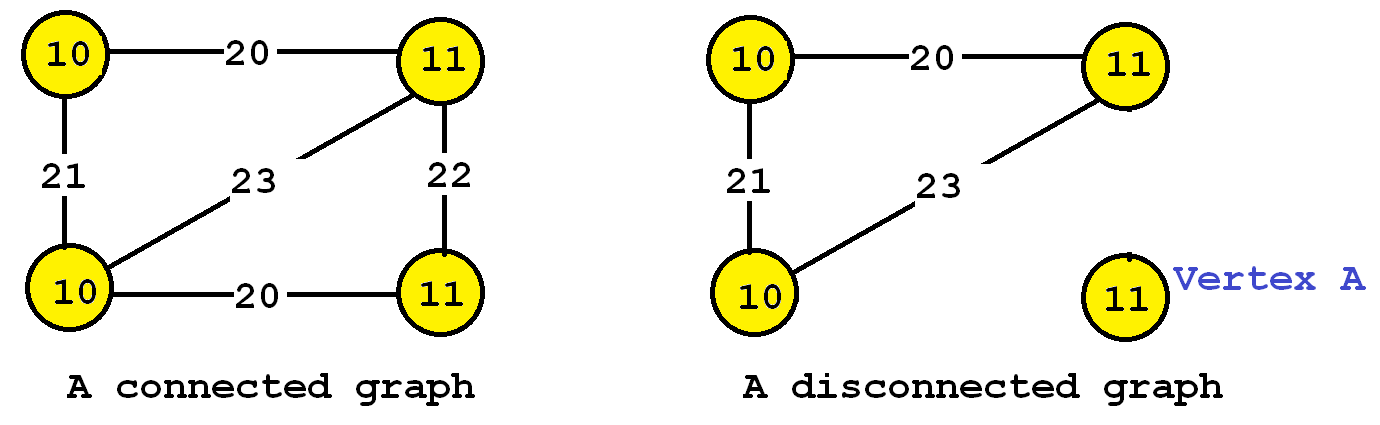The graph on the left is said to be a connected graph because by following the edges, it is possible to go from any vertex to any other vertices. For example, imagine that vertices represents cities and that the edges are roads between cities. A connected graph in this context is a graph where it is possible to go from any city to any other cities by following the roads.  If a graph is not connected, it is said to be a disconnected graph. For example, the graph on the right is disconnected since Vertex A cannot be reached from the other vertices by following the edges. In the following, we will use the term “graph” to refer to connected graphs.  Thus, all the graphs that we will discuss in the following paragraphs will be connected graphs.

Types of graphs: directed and undirected

It is also useful to distinguish between directed and undirected graphs. In an undirected graph, edges are bidirectional, while in a directed graph, the edges can be unidirectional or bidirectional. Let’s illustrate this idea with an example.The graph on the left is undirected, while the graph on the right is directed. What are some real-life examples of a directed graph?  For example, consider a graph where vertices are locations and edges are roads. Some roads can be travelled in both directions while some roads may be travelled in only a single direction  (“one-way” roads in a city).

Some data mining algorithms are designed to work only with undirected graphs, directed graphs, or support both.

Analyzing graphs

Now that we have introduced a bit of theory about graphs, what kind of data mining task can we do to analyze graphs?  There are many  answers to this question. The answer depends on what is our goal but also on the type of graph that we are analyzing (directed/undirected, connected/disconnected,  a single graph or many graphs).

In this blog post, I will explain a popular task called frequent subgraph mining. The goal of subgraph mining is to discover interesting subgraph(s) appearing in a set of graphs (a graph database). But how can we judge if a subgraph is interesting?  This depends on the application. The interestingness can be defined in various ways. Traditionally, a subgraph has been considered as interesting if it appears multiple times in a set of graphs. In other words, we want to discover subgraphs that are common to multiple graphs. This can be useful for example to find association between chemical elements common to several chemical molecules.

The task of finding frequent subgraphs in a set of graphs is called  frequent subgraph mining.  As input the user must provide:

• a graph database (a set of graphs)
• a parameter called the minimum support threshold (minsup).

Then, a frequent subgraph mining algorithm will enumerate as output all frequent subgraphs. A frequent subgraph is a subgraph that appears in at least minsup graphs from a graph database.  For example, let’s consider the following graph database containing three graphs: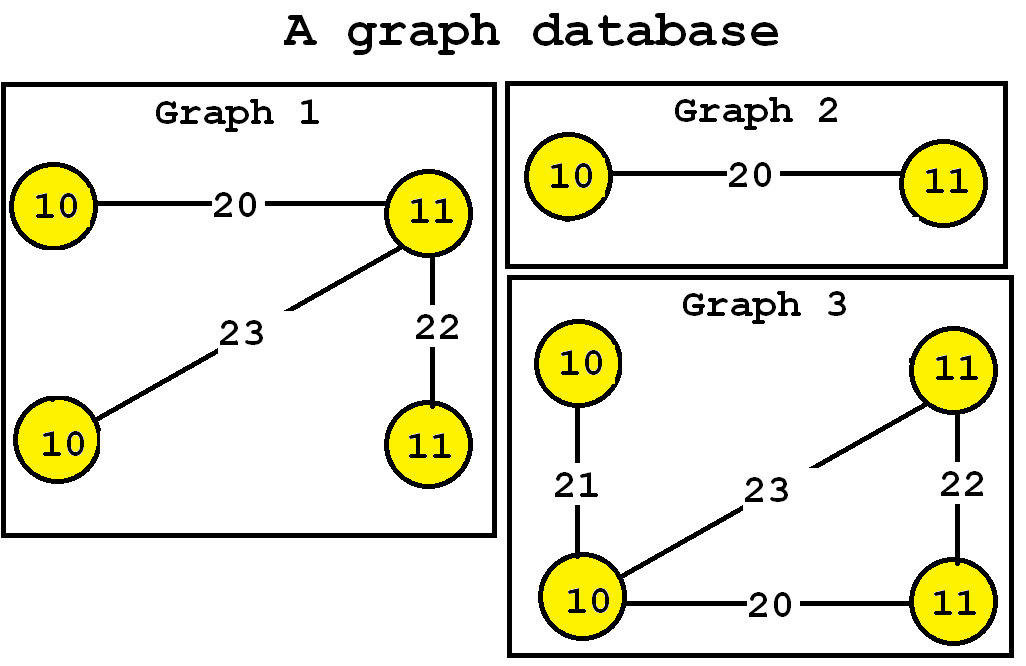Now, let’s say that we want to discover all subgraphs that appear in at least three graphs. Thus, we will set the minsup parameter to 3. By applying a frequent subgraph mining algorithm, we will obtain the set of all subgraphs appearing in at least three graphs:Consider the third subgraph (“Frequent subgraph 3”).  This subgraph is frequent and is said to have a support (a frequency) of 3 since it appears in three of the input graphs. These occurrences are highlighted in red, below: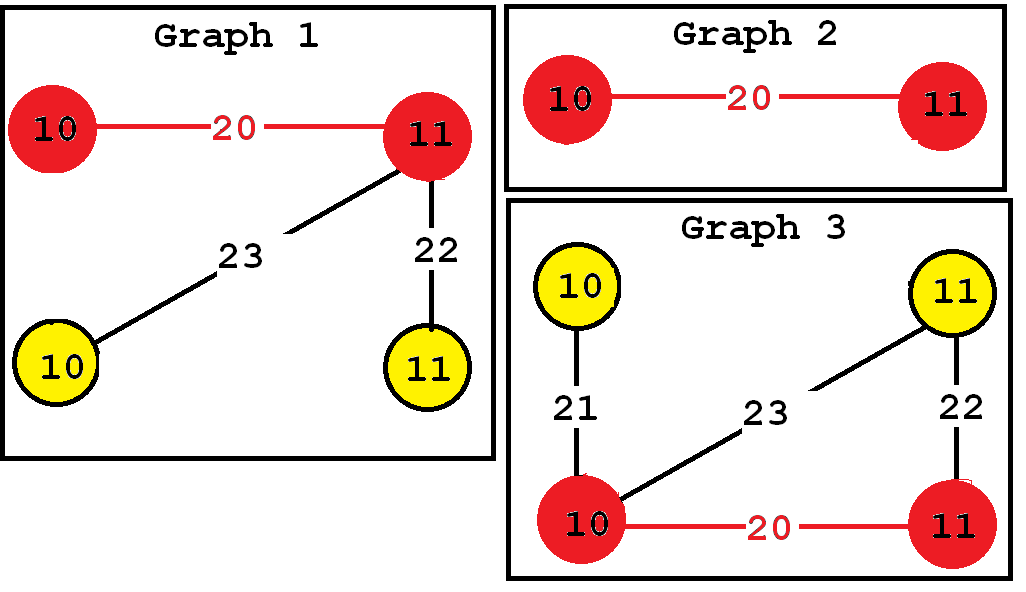Now a good question is how to set the minsup parameter? In practice, the minsup parameter is generally set by trial and error.  If this parameter is set too high, few subgraphs will be found, while if it is set too low, hundred or millions of subgraphs may be found, depending on the input database.

Now, in practice, which tools or algorithms can be used to find frequent subgraphs? There exists various frequent subgraph mining algorithms. Some of the most famous are GASTON, FSG, and GSPAN.

Mining frequent subgraphs in a single graph

Besides discovering graphs common to several graphs, there is also a variation of the problem of frequent subgraph mining that consists of finding all frequent subgraphs in a single graph rather than in a graph database. The idea is almost the same. The goal is also to discover subgraphs that appear frequently or that are interesting. The only difference is how the support (frequency) is calculated. For this variation, the support of a subgraph is the number of times that it appears in the single input graph. For example, consider the following input graph: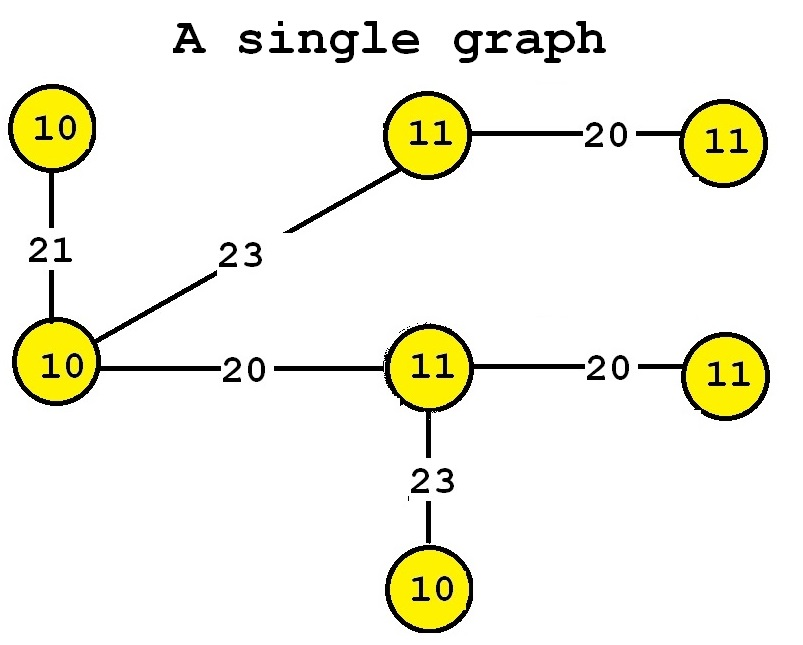This graph contains seven vertices and six edges. If we perform frequent subgraph mining on this single graph by setting the minsup parameter to 2, we can discover the five following frequent subgraphs: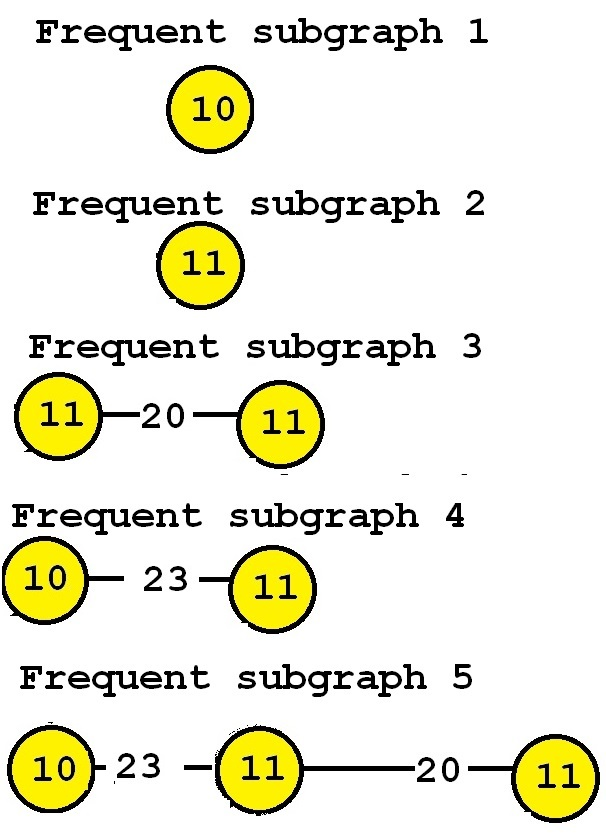These subgraphs are said to be frequent because they appear at least twice in the input graph. For example, consider “Frequent subgraph 5”. This subgraph has a support of 2 because it has two occurrences in the input graph. Those two occurrences are highlighted below in red and blue, respectively.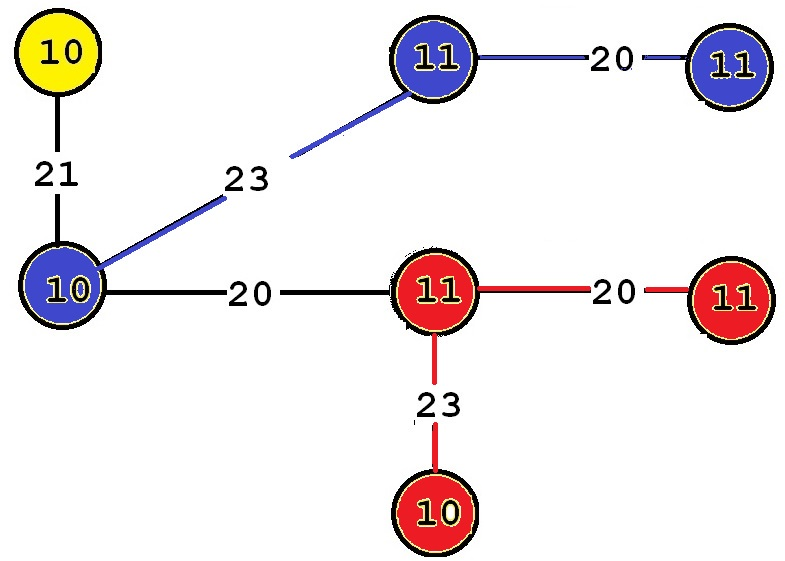Algorithms to discover patterns in a graph database can often be adapted to discover patterns in a single graph.

Want to try frequent subgraph mining?

If you want to try frequent subgraph mining algorithms, some public fast Java open-source implementations of TKG for top-k frequent subgraph mining and gSpan are available in the SPMF data mining library.

Conclusion

In this blog post, I have introduced the problem of frequent subgraph mining, which consists of discovering subgraphs appearing frequently in a set of graphs.  This data mining problem has been studied for more than 15 years, and many algorithms have been proposed.  Some algorithms are exact algorithms (will find the correct answer), while some other are approximate algorithms (do not guarantee to find the correct answer, but may be faster).

Some algorithms are also designed to handle directed or undirected graphs, or mine subgraphs in a single graph or in a graph database, or can do both. Besides, there exists several other variations of the subgraph mining problem such as discovering frequent paths in a graph, or frequent trees in a graph.

Besides, in data mining in general, many other problems are studied related to graphs such as optimization problems, detecting communities in social networks, relational classification, etc.

In general, problems related to graphs are quite complex compared to some other types of data. One of the reason why subgraph mining is difficult is that algorithms typically need to check for “subgraph isomorphisms“, that is to compare subgraphs to determine if they are equivalent. But nonetheless, I think that these problems are quite interesting as there are several research challenges.

I hope that you have enjoyed this blog post. If there is some interest about this topic, I may do another blog post on graph mining in the future.

Philippe Fournier-Viger is a professor of Computer Science and also the founder of the open-source data mining software SPMF, offering more than 120 data mining algorithms.

(Visited 5,292 times, 7 visits today)

### Related posts:

#### An introduction to frequent subgraph mining — 29 Comments

1.Dang Nguyen on said:

Hi Philippe,
Thank you for your time and effort to write this post. In my opinion, mining frequent subgraphs is an interesting and challenging topic since it requires more complex steps during mining process such as graph isomorphism checking that wasn’t mentioned in your post.
In “Mining frequent subgraphs in a single graph”, I think the frequent subgraph 4 should be 10-23-11.
HAPPY LUNAR NEW YEAR
Dang

• Hi Dang,

Glad that to know that you are still reading and commenting the blog. 🙂 And thanks for reporting the error about 10-23-11. I have now fixed it. Yes, I will modify the blog post to briefly mention isomorphism checking. It is a good idea. If I remember well, you had previously worked on graph mining. By the way, hope that everything is going well and also wish you a happy lunar new year. 🙂

Best,
Philippe

2.Dang Nguyen on said:

Thanks Philippe.

Yes, I used to work on frequent subgraph mining and published two papers on this topic. The main idea is to implement parallel versions of gSpan on multi-core processor computers. Recently, I have seen some works developing algorithms on distributed systems using Map-Reduce.

Best,
Dang

• Interesting. I have been reading on subgraph mining recently. I have implemented some existing algorithm for subgraph mining in Java for SPMF, recently. But I have unfortunately found that the algorithm was theoretically incorrect, so I have not released the source code.

I think that subgraph mining is interesting. I will probably try to write a paper about it when I can find some time. But now, I am just at the stage of looking for some ideas, to see if I can get some good idea about that topic.

Best,

Philippe

3.Khin Myat Kyu on said:

Hi Sir Philippe,
Thank you for your time and effort to write this post. I am doing research on graph based indexing. Firstly, I try to retrieve frequent subgraphs and build the indexing method using them. Your blog is very helpful to me. I am looking forward your next blogs.

Best regards,
Ms. Khin Myat Kyu

• Hello,
Thanks for your comment. It seems like a very interesting topic. I have seen some related idea before for sequences. There was a paper several years ago called SeqIndex, where they used to find sequential patterns in sequences to index a set of sequences. In case you don’t know about that paper, you may want to have a look. But I guess that graphs has many other challenges, so it should be an interesting project!
Best regards,

Philippe

•Khin Myat Kyu on said:

Dear Sir,
I am a Ph.D student in Myanmar. My research focus on indexing scheme that is based on frequent subgraphs which are mined from RDF data. I am ambiguous which one is the first process for me i.e., extracting frequent subgraphs from the RDF dataset or grpah representation such as cannonical labeling. Please kindly give me your suggestions!

Best regards,
Khin

• Hello, I did not work on graph indexing. But my assumption would be that you would need to extract patterns from the graphs, and then use these patterns as the index the search for the graphs. Thus, the patterns would act as a kind of key to quickly find the indexed graphs. So I assume that you would need some graph mining algorithm that can discover the frequent subgraphs and give you the list of graphs containing each patterns. Then, after that, you would need to build the index and write a retrieval algorithm to search for the graphs using that index. I think that is how it could work. Best regards

4.Elife on said:

Hi Sir Philippe,

Thank you for your effort to write this post. I am a Master student and I am working on Subgraph Mining. Do you know that which algorithms work with disconnected graph data as input dataset? I mean if we have two graphs as input but both of them are disconnected graphs and we want to find all subgraphs, which algorithm is the best? I would be appreciated if you answer my question. Thank you for your time and response.

Best,
Elife

• Hi,

Glad you like the post. I think subgraph mining is a very interesting topic but I am also quite new to this topic, so my answer may not be accurate. I think that most subgraph mining algorithm will find patterns that are connected but the input graph(s) does not need to be connected. So in other words, I think that the restriction of being connected only apply to the patterns that are found rather than the input graph(s).

Best,

Philippe

5. Dear Sir,

I need a graph dataset of Facebook or any other Social Media Web Site.

I need different graphs , in which each graph represent an individual user details.

Can you please guide me from where i can get such graph database having different small graphs in it, so that i can apply my algorithm to find all the frequent subgraph from this graph database

Thanks

6. Dear sir.
Thank you for your time and effort to write this post. I am Ph.D Student in Iran. I am doing research on frequent subgraph mining from single large graph as Ph.D thesis. My research focus on parallel mining frequent subgraph from single large graph. I need a advisor in parallel computing specially map reduce frame work or graph mining area. Can you guide me in this area?
Best regards,
Iman

• Dear Iman,
Glad you like the post. Graph mining is a good research area for doing your thesis. I am personally not very familiar with Map Reduce, and cannot really recommend an advisor related to that. I think that the most important is to first master the concept of MapReduce, and then to see how you can transfer concepts of graph mining to Map Reduce to create a new algorithm. So I would suggest to first read on map reduce and do some programming examples. Then, to read about graph mining, and especially map reduce algorithms for graph mining if there are some already. You could then start by implementing some know algorithms and then think about how to improve them.

Best regards,
Philippe

7. Respected Sir,

This is very good Introductory Chapter, you shared. Thanks

I am working on Frequent Subgraph Mining. I need a graph dataset of Facebook or any other Social Media Web Site having multiple graph (i.e. i need social media transnational graph dataset)

If you have such dataset then share to me OR Can you please guide me from where i can get such graph database having different small graphs in it, so that i can apply my algorithm to find all the frequent subgraph from this graph database

Thanks

8.Shreya on said:

Thank you for writing this article. I was having a hard time understanding why the vertice labels could be non-unique and was wondering what kind of real life scenario such graphs would apply to. This really cleared it up for me!

9.sakura on said:

thanks for this awesome explanation

10.Azar on said:

is there any predefined tool that we can use to find the frequent graphs?

• There are some source code and executables available on GitHub and other websites. If you search some algorithms like GSpan and Gaston, you can find the executables or source code on some websites. However, I did not find any software that provide many algorithms. Usually, it is just some authors that provide an implementation of one algorithm and that’s all. By the way, soon, I will release a fast Java implementation of gSpan as part of my SPMF data mining software (I am cleaning the code currently).

11. Thank you for the tutorial!

What will be great is to develop the SPMF in Python too. I would be happy to help if this is of interest.

Sincerely

• Dear Ahmed,

Thanks for reading the tutorial and your message. Yes, I think Python now is becoming more and more popular so it would be good to have something for Python. However, converting all the code of SPMf to Python would take years maybe since there are about 150 algorithms, and some are quite complex. I think a better way could be to make a wrapper around SPMF to be able to call it from Python. A few years ago someone made a mini wrapper to call the VMSP algorithm of SPMF from Python ( https://github.com/fandu/maximal-sequential-patterns-mining ). If we could make a more general wrapper that could call any SPMF algorithm, it would be great. I think it should not be too hard to do because SPMF is well standardized… It can be called from the command line and all algorithms are described by a Java class with their parameters, and SPMF take some text file as input and produce a text file as output whicih can interact easily with other language. But I am not familiar with Python and I am quite busy, so I will likely not do it by myself. I would need a bit of help 😉

Best regards,
Philippe

12.Jasmin Ara on said:

I am novice at frequent graph mining and so feeling difficulty in understanding this work.
I would be grateful enough if you help me a little.
How can I represent the graph by adjacency list where each node label is not distinct and edges are also not distinct??

• Hi,
I think that a simple solution is to give a unique identifier to each node and each edge. For example, you can call the nodes: node1, node2, node3 … and the edges: edge1, edge2…
Then, when you build the adjacency list, you would use the identifiers which are unique instead of using the labels which may not be unique.

I think that this could be one very simple way to solve the problem. But I did not read much about adjacency list. Maybe there are also some other way.

Best regards,

Philippe

•Jasmin Ara on said:

Dear Philippe,